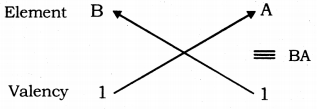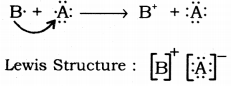# Consider two elements ‘A’ (Atomic number 17) and ‘B’ (Atomic number 19)

Consider two elements ‘A’ (Atomic number 17) and ‘B’ (Atomic number 19).
(i) Write the positions of these elements in the modern periodic table giving justification
(ii) Write the formula of the compound formed when ‘A’ combines with ‘B
(iii) Draw the electron dot structure of the compound and state the nature of the bond formed between the two elements.

(i) Position of the elements in the periodic table:

Element Period Group
A 3 17
B 4 1

(ii) Atomic number of A = 17 ; Electronic configuration A = 2, 8, 7
Number of valence electrons of A = 7 ; Valency ofA = 8 - 7= 1
Atomic number of B = 19 ; Electronic configuration B = 2, 8, 8, 1
Number of valence electrons of B = 1; Valency of A = 1So, the formula of the compound formed when elements A and B combine is BA.

(iii)